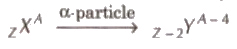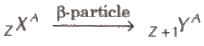# Radioactivity - Notes | Study Physics Class 12 - NEET

 1 Crore+ students have signed up on EduRev. Have you?

The phenomena of disintegration of heavy elements into comparatively lighter elements by the emission of radiations is called radioactivity. This phenomena was discovered by Henry Becquerel in 1896.

(i) α-rays

(ii) β-rays

(iii) γ – rays

α-rays consists of α-particles, which are doubly ionised helium ion.

β-rays are consist of fast moving electrons.

γ – rays are electromagnetic rays.

When an α – particle is emitted by a nucleus its atomic number decreases by 2 and mass number decreases by 4.When a β -particle is emitted by a nucleus its atomic number is Increases by one and mass number remains unchanged.When a γ – particle is emitted by a nucleus its atomic number and mass number remain unchanged

The rate of disintegration of radioactive atoms at any instant is directly proportional to the number of radioactive atoms present in the sample at that instant.

Rate of disintegration (– dN / dt) ∝ N

– dN / dt = λ N

where λ is the decay constant.

The number of atoms present undecayed in the sample at any instant N = No e-λt

where, No is number of atoms at time t = 0 and N is number of atoms at time t.

The time is which the half number of atoms present initially in any sample decays, is called half-life (T) of that radioactive element.

Relation between half-life and disintegration constant is given by

T = log2e / λ = 0.6931 / λ

Average Life or Mean Life(τ)

Average life or mean life (τ) of a radioactive element is the ratio of total life time of all the atoms and total number of atoms present initially in the sample.

Relation between average life and decay constant τ = 1 / λ

Relation between half-life and average life τ = 1.44 T

The number of atoms left undecayed after n half-lives is given by

N = No (1 / 2)n = No (1 / 2) t/T

where, n = t / T, here t = total time.

The activity of a radioactive element is equal to its rate of disintegration.

Activity R = (– dN / dt)

Activity of the sample after time t,

R = Ro-λt

Its SI unit is Becquerel (Bq).

Its other units are Curie and Rutherford.

1 Curie = 3.7 * 1010 decay/s

1 Rutherford = 106 decay/s

The document Radioactivity - Notes | Study Physics Class 12 - NEET is a part of the NEET Course Physics Class 12.
All you need of NEET at this link: NEET

## Physics Class 12

157 videos|452 docs|213 tests

## Physics Class 12

157 videos|452 docs|213 tests

### How to Prepare for NEET

Read our guide to prepare for NEET which is created by Toppers & the best Teachers

Track your progress, build streaks, highlight & save important lessons and more!

,

,

,

,

,

,

,

,

,

,

,

,

,

,

,

,

,

,

,

,

,

;